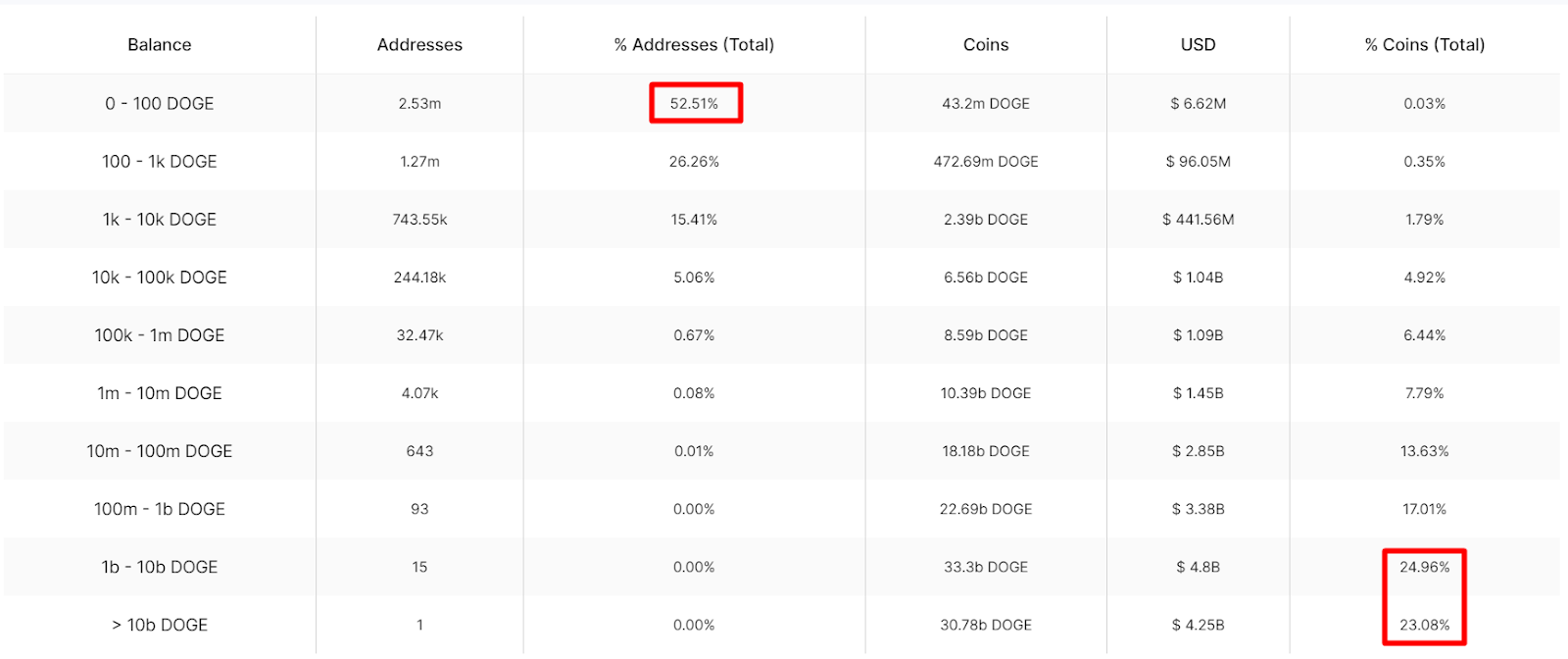# Holdings Distribution Matrix

## Overview

The Holdings Distribution Matrix indicator shows a breakdown of different groups of addresses according to the balance that they hold.
The first column (Balance) is the balance group, meaning that each data on that row will refer to addresses that have a balance of that cryptocurrency between the ranges of that group. The second column (Addresses) counts the total number of addresses that have some balance between the specified ranges in the first column. The third column (% Addresses Total) shows which percentage over the total number of addresses with balance represents each group.
Coins is the fourth row and shows the total number of cryptocurrencies held by the selected group. The fifth row (USD) converts the value of the total balance of each group to USD according to the last price of the cryptocurrency. At the end, the sixth column represents the total amount of the supply that each group has.

## 💡 How can I use it?Holdings Distribution Matrix of DOGE (Dogecoin)
The Holdings Distribution Matrix is very useful to glimpse how much of the supply is held by whales or small investors.
As can be seen in the example above, for DOGE more than half of the addresses with balance hold between 0 and 100 DOGE, which would be balances with a maximum around \$12. Although these addresses are the majority, at current prices the sum of those balances would be \$6.62M. While on the other hand there is a single address that holds \$4.25B in DOGE alone, accounting for more than 23% of the supply.
If the next richest 15 addresses are counted (balance between 1b to 10b DOGE) then their supply would be almost 50% of the total supply of DOGE. Coins with supply very concentrated could potentially be considered riskier for some investors due to the selling pressure that could arise if the few whales holding most of the supply would decide to sell their coins.